# Implementation of Stack using Array in C

## Implementation of Stack using Array in C:

In this article, I am going to discuss the Implementation of Stack using Array in C Language with Examples. Please read our previous article where we give you an introduction to the Stack Data Structure.

##### How to Implement Stack using Array in C?

We have already seen Stack ADT in the previous article, where we saw that for the definition of the stack, we require data representation and the operations on the data. So first of all, we need the representation of data. For implementing a stack, what are the things required when we are using an array? Let us understand this with an example.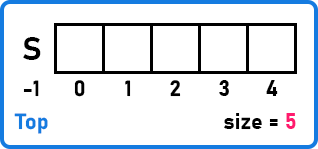Here, we have taken an array of size 5. Also, we have a variable i.e. size where we are storing the size of the array. Next, we need a top pointer that will be pointing to the topmost element of the array. So, here we have taken a variable called Top that will store the topmost element of the array.

###### What should be the data type of the Top pointer?

Integer, because it is pointing on the index. So, Top will point to the recently inserted element in the array.

###### What should be the data type of the array?

This depends on what type of values you are storing in the array. Here we have taken an integer array.

So, we need 3 things, an array of some size, the size of the array, and the topmost element of the array. That means we need 3 things for implementing a stack by using an array in C Language.

Inside the stack, we are inserting and deleting the element from the same end. Insertion and deletion will be done from the top. An array has two ends. We can insert or delete from any end of the array (right or left end).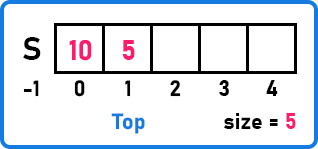Here we have a few elements in the array i.e. 10 and 5 which are stored at index positions 0 and 1 respectively. Now we want to insert one more element which is 2. So where should we insert it? At index 0 or at index 2? If we are inserting at index 0, then we have to shift all the elements to the right-hand side. So, inserting at index 2 will be a better way. So, we are inserting and deleting from the right-hand side of the array as shown in the below image.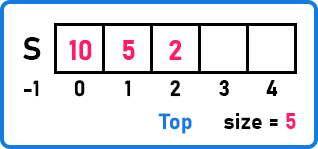Insertion and deletion from the right side will take constant time (O(1)). We have updated the Top pointer to index 2 which is pointing to element 2. When we are working with a stack, we usually imagine the stack is vertical as shown in the below image. In recursion, we have seen that the stack was vertical.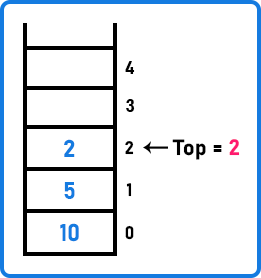This is the representation of the array as a stack. We will represent the array as a vertical lane or stack. So, we require 3 things (array, size, and top). So, for this, we will combine them together and define a structure. We can define a structure by grouping the related things under one name. So let us define a structure for the stack as follows.

```struct Stack {
int size;
int top;
int *A;
};
```

Here, we have the size and top variables of the type integer. And we have not declared any size for the array. Because the size of the array will be declared at runtime of the program. So, at runtime, an array will be created with a user-defined size. We have taken a pointer in our structure. So, with the pointer, we will create an array at runtime, so that we can dynamically create a stack. We are working with integer data so we have taken integer-type pointer in our structure.

We will be using this structure wherever we will be using a stack. Now let us see how to create and initialize this stack within the main method.

```int main(){
struct Stack st;
printf("Enter size of Stack: ");
scanf("%d", &st.size);
st.A = (int *)malloc(sizeof(int) * st.size);
st.top = -1;
}
```

Inside the main function, we have created an object st of the structure Stack.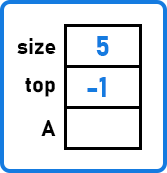Now, once the stack st is created, we will get an object that is st having 3 things inside it, which are size, top, and A (pointer). Now for creating a stack dynamically, we should know the size of the stack. So let us take the size as input from the user. Suppose we got a size of 5 from the user. Now we know the size, so we will create an array of size 5 as shown in the below image.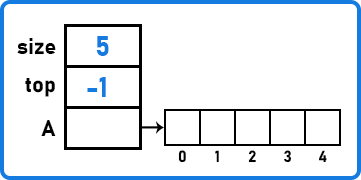Now, one more thing we have to do. We know that the top will point to the topmost element. For now, the stack is empty. So, there is no topmost element. Then where the top should point to? It should point outside the stack. It means if there is no element, the top should point outside the stack and if there is an element then point to the recently inserted element. The first element will be stored at index 0 in the array. So the top should point to -1 if there is no element. So, we can create a stack with the above set of statements in C language. We are using the entire thing (stack) as a structure.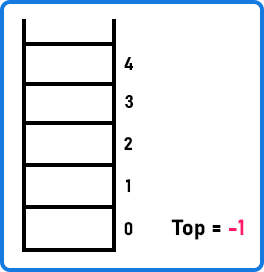We will be looking at this diagram when we are working with the stack. Now, two important things here.

###### When will you say that the stack is empty?

Like now the stack is empty. So, what is the condition? When the top is at -1 then the stack is empty.

Condition for Empty Stack: if (top == -1)

###### When we can say that the stack is full?

If the top is pointing to a size-1 location the stack will be full.

Condition For Full Stack: if (top == (size-1))

In the next article, we will be looking at how we will perform the operations on the stack. Here, in this article, I try to explain, how to Implement a Stack using an Array in C Language with Examples. I hope you enjoy this Stack Implementation using an Array in C Language article.